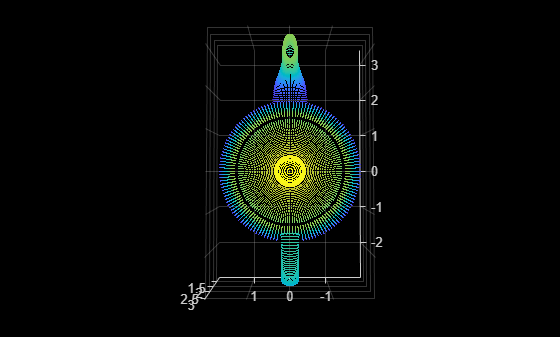# removeHiddenPoints

Remove hidden points from point cloud

Since R2023a

## Syntax

``ptCloudOut = removeHiddenPoints(ptCloudIn,viewPoint)``
``ptCloudOut = removeHiddenPoints(ptCloudIn,viewPoint,RadiusScale=rScale)``
``[ptCloudOut,indices] = removeHiddenPoints(___)``

## Description

example

````ptCloudOut = removeHiddenPoints(ptCloudIn,viewPoint)` removes hidden points from the point cloud `ptCloudIn`. The function removes the points hidden when viewing the point cloud from the specified viewpoint `viewPoint`. Determining the visibility of a point can be useful for shadow casting, reconstruction, and camera placement.```
````ptCloudOut = removeHiddenPoints(ptCloudIn,viewPoint,RadiusScale=rScale)` specifies the radius scale of the spherical projection.```
````[ptCloudOut,indices] = removeHiddenPoints(___)` returns the indices of the points visible from the specified viewpoint, using any combination of input arguments from previous syntaxes.```

## Examples

collapse all

Read point cloud data from a PLY file into the workspace.

`ptCloud = pcread("teapot.ply");`

Define the viewpoint.

`viewPosition = [0 0 13];`

Compute the indices of the visible points in the point cloud from the specified viewpoint, and remove the hidden points.

`[ptCloudOut,indices] = removeHiddenPoints(ptCloud,viewPosition);`

Display the visible points.

```pcshow(ptCloudOut) campos(viewPosition)```## Input Arguments

collapse all

Input point cloud, specified as a `pointCloud` object.

Viewpoint, specified as a three-element vector of the form [x y z]. Viewpoint can be inside or outside the point cloud.

Data Types: `single` | `double`

Radius scale of the spherical projection, specified as a positive scalar. The function scales the radius to a value of `10n`, where n is the value of `rScale`. Increasing the radius scale increases the number of visible points.

Note

Specify a higher radius scale value for dense point clouds.

Data Types: `single` | `double`

## Output Arguments

collapse all

Output point cloud, returned as a `pointCloud` object.

Indices of the points visible from the specified viewpoint, returned as an M-element column vector. M is the number of visible points.

## Algorithms

The function uses these steps to determine the visible points in a point cloud from a specified viewpoint.

1. Associate the point cloud with a coordinate system whose center lies at the viewpoint.

2. Perform inversion using spherical projection.

1. Create a sphere of radius R such that all points in the point cloud lie within the sphere. You can control the radius value by using the `rScale` input.

2. Transform the point cloud by reflecting each point, with respect to the sphere, along the line joining the point and the viewpoint.

3. Calculate a convex hull of the transformed point cloud and the viewpoint. The points inside the convex hull are the visible points.

## Version History

Introduced in R2023a